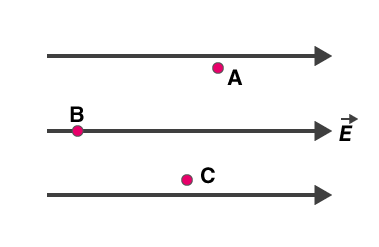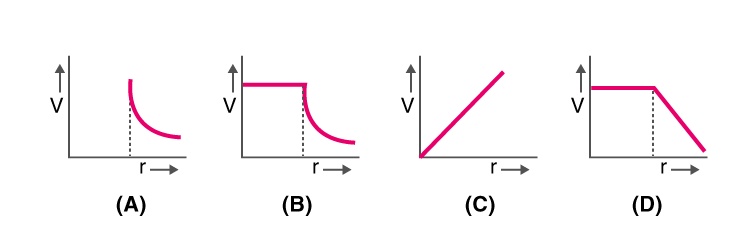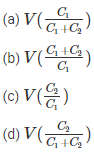# Electro statistics MCQ for NEET

NEET  Physics is the scoring paper in the medical entrance examination. Here, you will discover the NEET Physics MCQ Questions for all Concepts as per the latest syllabus. Practice more on a regular basis with these NEET Physics objective questions on air pollution and improve your subject knowledge & problem-solving skills along with time management. NEET Physics Electro statistics Multiple Choice Questions make you feel confident in answering the question in the exam & increases your scores to high.

## MCQ on Electro statistics

1. What will be the flux coming out of any surface a cube, if a change QμC is placed at the centre of the cube?Answer: (d) $$\frac{Q}{6 c_{0}} \times 10^{-6}$$

2. Name the law that states the force between electric charges
(a) Ohm’s law
(b) Coulomb’s law
(d) Ampere’s law

3. What happens to the force acting between the charged particles, if the distance between these charged particles is halved?
(a) It increases by four times
(b) It gets doubled
(c) It becomes half
(d) It reduces by one-fourth

Answer: (a) It increases by four times

4. What is the electric potential of the points A, B, and C which are in a uniform electric field?(a) The electric field is the same at all three points A, B, and C
(b) Maximum at A
(c) Maximum at B
(d) Maximum at C

5. What is the minimum charge on a particle?
(a) 1 Coulomb
(b) 1.6 x 10-19 Coulomb
(c) 3.2 x 10-19 Coulomb
(d) 6.6 x 10-19 Coulomb

Answer: (b) 1.6 x 10-19 Coulomb

6. For a hollow spherical shell, potential, V changes with respect to distance, r from the centre. Which graph from the following represents this?7. What is the graphical representation of an electric field due to the uniformly charged sphere of radius R which is a function of distance from the centre?8. The capacity of parallel plate condenser is dependent on the
(a) The separation between the plates
(b) The metal used for the construction
(c) The thickness of the plate
(d) The potential applied across the plates

Answer: (a) The separation between the plates

9. The mathematical representation of the force of attraction between the plates of the parallel plate capacitor is given asAnswer: (c) $$\frac{q^{2}}{2 \epsilon_{0} A K}$$

10. What does an electric dipole experience when it is kept in the non-uniform electric field?
(a) Only a force
(b) Only torque
(c) Force and torque both
(d) Neither force nor torque

Answer: (c) Force and torque both

11. The capacitance of the capacitor is independent of
(a) The charges present on the plate
(b) The distance of separation between the plates
(c) The shape of the plates
(d) The size of the platesAnswer: (d) $$V\left(\frac{C_{2}}{C_{1}+C_{2}}\right)$$